# Important 4 Marks Questions for CBSE 9 Maths

Important 4 marks questions for CBSE Class 9 Maths are available here with solutions. All the questions are prepared here as per the latest exam patterns and syllabus (2022-2023). Class 9th is a crucial phase in the life of students as this form the basis for next higher classes. CBSE conducts examinations for the schools affiliated with the central board. Students usually find Maths as one of the most challenging subjects. The main reason for their fear arises due to a lack of confidence & practice for the subject. If one wishes to excel in an examination, it is quite essential to practice questions daily.

We at BYJU’S provide students of class 9th with important 4 marks questions and solutions to practice and to be aware of the type of questions asked in the final exam.

## Class 9 Maths 4 Marks Important Questions

Question 1- Water flows in a tank 150m

$$\begin{array}{l}\times\end{array}$$
100m at the base through a pipe whose cross-section is 2 dm
$$\begin{array}{l}\times\end{array}$$
1.5 dm at the speed of 15 km/h. In what time, will the water be 3m deep?

Solution:

Volume of water in the tank when depth of the tank is 3 m will be:

V = 150 × 100 × 3 = 45000 m³

Rate of volume of water flowing = 2 × 1.5 × 10-2 × 15000 m³/hr

= 450 m³/hr

Required time = (Volume of water in the tank)/ (Volume of water flowing through the pipe per hour)

= 45000/450

= 100 hours

Question 2- A solid cylinder has a total surface area of 462 sq. cm. Its curved surface area is one-third of its total surface area. Find the volume of the cylinder. (Assume

$$\begin{array}{l}\pi = \frac{22}{7}\end{array}$$
).

Solution:

Given,

Total surface area of a solid cylinder (TSA) = 462 sq.cm

i.e. 2πr(r + h) = 462 sq.cm

And,

CSA = (1/3) TSA

2πrh = (1/3) 462 sq.cm

2πrh = 154 sq.cm….(i)

From the given,

2πr(r + h) = 462 sq.cm

2πr² + 2πrh = 462 sq.cm….(ii)

From (i) and (ii),

2πr² + 154 = 462

2πr² = 308

r² = 308/2π

r² = 308 × 1/2 × 7/22

r² = 28 × 1/2 × 7/2

r = √49

r = 7 cm

Now,

2πrh = 154

h = 154/2πr

h = 154 × 1/2 × 7/22 × 1/7 cm

h = 7/2 cm

Volume of cylinder = πr²h

= 22/7 × 7² × 7/2 cm³

= 539 cm³

Question 3- A recent survey found that the age of workers in a factory as follows:

 Age (in years) 20-29 30-39 40-49 50-59 60 and above Number of Workers 38 27 86 46 3

If a person is selected at random, then find the probability that the person is:

(i) Having age from 30-39 years.

(ii) Under 40 years.

(iii) 40 years or more.

Solution:

Total number of workers = 38 + 27 + 86 + 46 + 3 = 200

(i) P(the person having age 30-39 years) = 27/200

(ii) P(the person having age under 40 years) = P(the person having age 20 – 29 years) + P(the person having age 30 – 39 years)

= (38/200) + (27/200)

= (38 + 27)/200

= 65/200

= 13/40

(iii) P(the person having 40 years or more) = P(the person having age 40 – 49 years) + P(the person having age 50 – 59 years) + P(the person having age 60 and above)

= (86/200) + (46/200) + (3/200)

= (86 + 46 + 3)/200

= 135/200

= 27/40

Question 4- If each diagonal of a quadrilateral separates it into two triangles of equal area, then show that the quadrilateral is a parallelogram.

Solution:

In quadrilateral ABCD, let us consider the diagonal AC.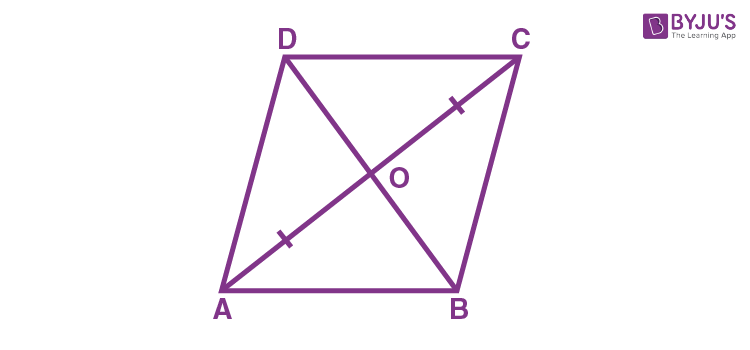ar(ΔAOB) + ar(ΔBOC) = ar(ΔAOD) + ar(ΔDOC)….(i)

Now, let us consider another diagonal BD.

Thus,

ar(ΔABD) = ar(ΔBCD)

ar(ΔAOD) + ar(ΔAOB) = ar(ΔBOC) + ar(ΔCOD)….(ii)

Subtracting (ii) from (i),

ar(ΔAOD) – ar(ΔBOC) = ar(ΔBOC) – ar(ΔAOD)

ar(ΔAOD) + ar(ΔAOD) = ar(ΔBOC) + ar(ΔBOC)

2 ar(ΔAOD) = 2 ar(ΔBOC)

ar(ΔAOD) = ar(BOC)

ar(ΔAOD) + ar(ΔAOB) = ar(ΔBOC) + ar(ΔAOB)

Since triangles ADB and ABC have the common base AB and lie between two lines AB and DC.

Therefore, AB is parallel to CD.

Similarly, AD is parallel to BC.

Hence, ABCD is a parallelogram.

Question 5- Hindustan metro company manufactures car batteries of a particular type. The life of 32 batteries (in years) was recorded as follows:

 2.8 3 3.7 3.2 2.9 4.1 3.5 4.5 3.5 2.8 3.2 3.4 3.8 3.2 4.6 3.7 2.7 4.4 3.4 3.3 2.9 3 4.3 3.5 3.5 3.2 3.9 3.2 3.2 3.1 3.7 3.4

(i) Find the probability that the life of a battery randomly select is less than or equal to 3 years.

(ii) If the company gives the warranty of a battery which is less than 4 years, then what is the probability of getting no complaints under the warranty period?

(iii) As per the given data, if the company gives a warranty of less than or equal to 2 years, then summit decides to purchase the battery. What his decision shows of getting no complaints under the warranty period?

(iv) Find the probability that the life of a battery randomly selected us less than or equal to 3.5 years.

Solution:

(i) Number of batteries with a life less than or equal to 3 years = 7

i.e. 2.8, 3.0, 2.9, 2.8, 2.7, 2.9, 3.0

Probability that the life of a battery randomly select is less than or equal to 3 years = 7/32

(ii) Number of batteries with life more than 4 years = 5

Probability of getting no complaints under the warranty period = 5/32

(iii) Number of batteries with lifetime more than 2 years = 32

That means, no battery would get complaints under warranty.

Hence, the probability of getting no complaints under the warranty period = 32/32 = 1

(iv) Number of batteries with life less than or equal to 3.5 years = 22

Therefore, the probability that the life of a battery randomly selected us less than or equal to 3.5 years = 22/32 = 11/16

Question 6- A well with 10 m inside diameter is dug 14 m deep. Earth taken out of it is spread all around to a width of 5 m to form an embankment. Find the height of the embankment.

Solution:

Given,

Inner diameter of the well = 10 m

Inner radius of the well = 10/2 = 5 m

Depth of the well = 14 m

We know that,

Volume of the cylinder = πr2h

= π × 52 × 14….(i)

From the given, tThe earth taken out from the well is evenly spread all around it to form an embankment.

Also, the width of the embankment = 5 m

So, the outer radius of the well = 5 + 5 = 10 m

Volume of the hollow embankment = π (R2 – r2) × h

= π × (102 – 52) × h….(ii)

By equating (i) and (ii),

π × 52 × 14 = π × (102 – 52) × h

h = (25 × 14) / 75

h = 14/3 m

Therefore, the height of the embankment so formed is 14/3 m.

Question 7- ABCD is a cyclic quadrilateral whose diagonals AC and BD intersect at P. If AB = DC, then prove that

(i)

$$\begin{array}{l}\Delta PAB \cong \Delta PDC\end{array}$$

(ii) PA = PD and PC = PB

$$\begin{array}{l}\parallel\end{array}$$
BC

Solution:

Given, ABCD is a cyclic quadrilateral such that AB = DC.

AC and BD are diameters.

AC and BD intersect each other at P.

Here, the centre of the circle and P are the same, i.e. O = P.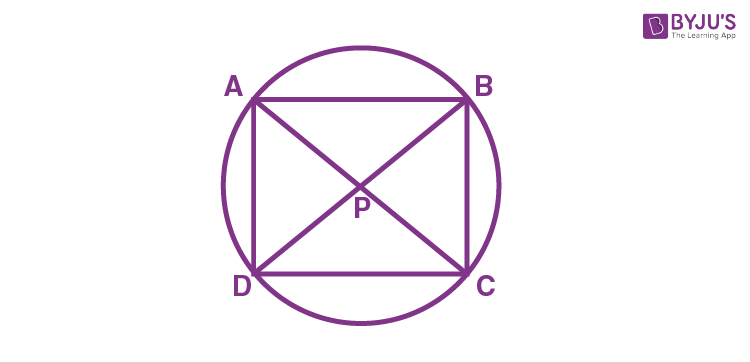In ΔPAB and ΔPDC,

OA = OD (radii of the same circle)

OB = OC (radii of the same circle)

∠AOB = ∠DOC (vertically opposite angles)

By SAS congruence rule,

ΔPAB ≅ ΔPDC

By CPCT,

PA = PD and PC = PB

As we know, diagonals are bisectors.

Thus, ABCD is a parallelogram.

Question 8- The difference between the outside and inside surfaces of a cylindrical metallic pipe 14 cm long is 44

$$\begin{array}{l}cm^{2}\end{array}$$
. If the pipe is made of 99 cu. cm of metal, find the outer and inner radii of the pipe.

Solution:

Let r and R be the inner and outer radius of a metallic pipe and h be its height.

Given,

The difference between the outside and inside surfaces of a cylindrical metallic pipe = 44 cm2

i.e. 2πRh – 2πrh = 44

2πh( R – r) = 44

2 × (22/7) × 14 × (R – r) = 44

R – r = 1/2 = 0.5….(i)

Also, given that the pipe is made up of metal= 99 cm³

That means,

Volume of cylindrical metallic pipe = πR2h – πr2h = 99

(22/7) x 14 (R2 – r2) = 99

44(R2 – r2) = 99

(R2 – r2) = 99/44 = 9/4

R2 – r2 = 2.25

(R – r)(R + r)  = 2.25

(0.5)(R + r) = 2.25  [from (i)]

R + r = 2.25 / 0.5

R + r  = 4.5….(ii)

2R = 4.5 + 0.5 = 5

R = 2.5 cm (outer radius)

Substituting R = 2.5 in (i),

r  = 2 cm (inner radius)

Question 9- In a

$$\begin{array}{l}\Delta PQR\end{array}$$
, find the measure of angles of the triangle formed by joining the mid-points of the sides of the triangle.

Solution:

Let P, Q and R be the midpoints of sides  AB, BC and AC respectively.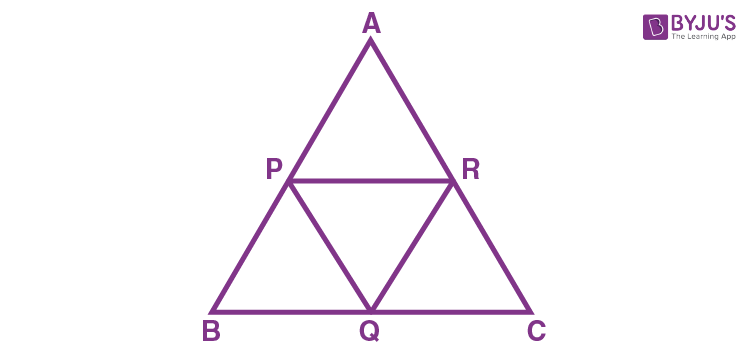Line segment joining the midpoints of two sides of the triangle is half of the third side.

That means,

PQ  = AC/2 ⇒ PQ/AC = 1/2

QR =  AB/2 ⇒ QR/AB = 1/2

PR  = BC/2 ⇒ PR/BC = 1/2

Thus, PQ/AC = QR/AB = PR/BC = 1/2

Therefore, ΔPQR ~ ΔACB   (corresponding sides are proportional)

We know that the corresponding angles of similar triangles are equal.

Therefore, ∠P = ∠A, ∠Q = ∠C, ∠R = ∠B

Question 10- Construct a triangle in which BC = 8 cm ,

$$\begin{array}{l}\angle B = 45^{\circ}\end{array}$$
and AB – AC = 3.5 cm.

Solution:

The steps to draw the triangle of given measurement is as follows:

1. Draw a line segment of base BC = 8 cm
2. Measure and draw ∠B = 45° and draw the ray BX
3. Take a compass and measure AB – AC = 3.5 cm.
4. With B as centre and draw an arc at the point be D on the ray BX
5. Join DC.
6. Now draw the perpendicular bisector of the line CD and the intersection point is taken as A.
7. Now join AC.
8. Therefore, ABC is the required triangle.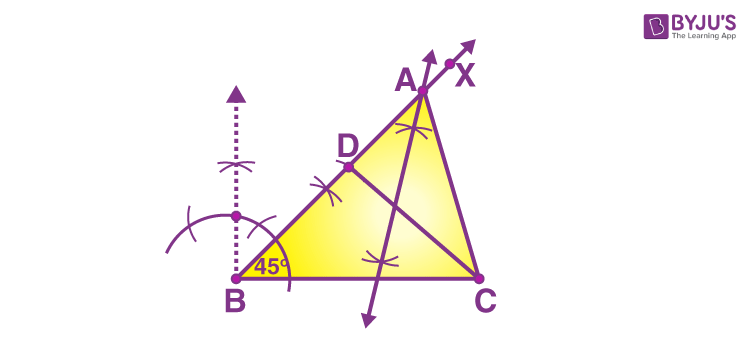Question 11- Construct the histogram and frequency polygon for the following frequency distribution:

 Weight(in kg) 40-45 45-50 50-55 55-60 60-65 65-70 Number of Persons: 12 25 28 15 12 5

Solution:

x-axis: Weight (in kg)

y-axis: Frequency, i.e. number of persons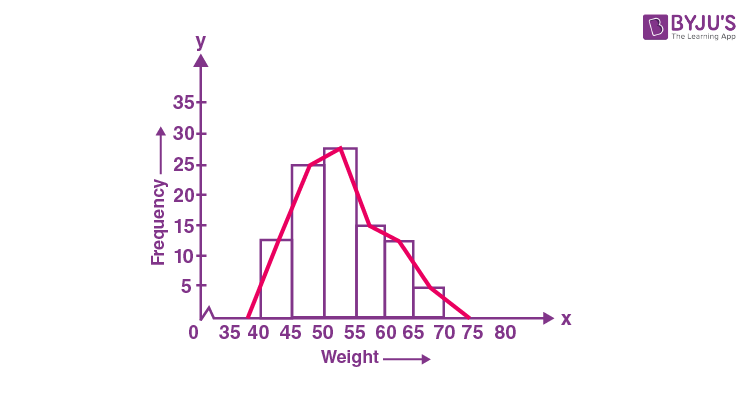Question 12- In Fig. given below, PQ is the diameter of the circle with centre O. If

$$\begin{array}{l}\angle PQR = 65^{\circ}, \angle RPS = 40^{\circ}\end{array}$$
and
$$\begin{array}{l}\angle PQM = 50^{\circ}\end{array}$$
, find
$$\begin{array}{l}\angle QPR, \angle PRS\end{array}$$
and
$$\begin{array}{l}\angle QPM\end{array}$$
.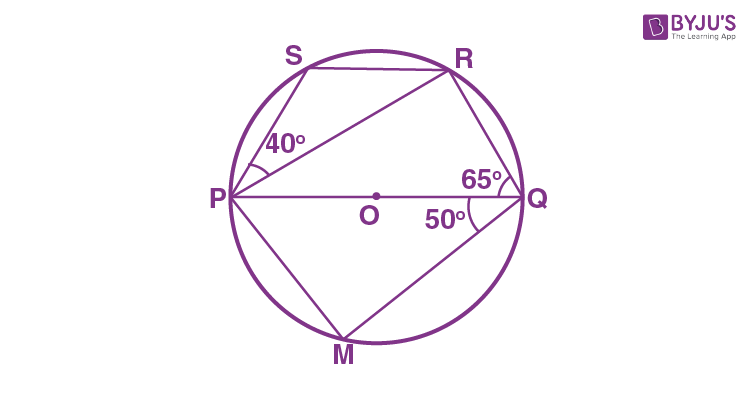Solution:

PQ is a diameter

∴ ∠PRQ = 90°  (since the angle in a semicircle is 90°)

In ΔPQR,  ∠QPR + ∠PRQ + ∠PQR = 180° (by the angle sum property of a triangle)

⇒ ∠QPR + 90° + 65° = 180°

⇒ ∠QPR + 155° = 180°

⇒ ∠QPR = 180° – 155°

⇒ ∠QPR = 25°

As we know, PQRS is a cyclic quadrilateral.

∴ ∠PSR + ∠PQR = 180°  (opposite angles of a cyclic quadrilateral are supplementary)

⇒ ∠PSR + 65° = 180°

⇒ ∠PSR = 180°- 65°

⇒ ∠PSR = 115°

In DPSR,  ∠PSR + ∠SPR + ∠PRS = 180°

⇒ 115° + 40° + ∠PRS = 180°

⇒ 115° + ∠PRS = 180°

⇒ ∠PRS = 180° – 155°

⇒ ∠PRS = 25°

From the given, PQ is a diameter.

∴ ∠PMQ = 90°

In ΔPMQ,

∠PMQ + ∠PQM + ∠QPM = 180°

⇒ 90° + 50° + ∠QPM = 180°

⇒ 140° + ∠QPM = 180°

⇒ ∠QPM = 180° – 140°

⇒ ∠QPM = 40°

Question 13- Draw the graph of linear equation x + 2y = 8. From the graph drawn, check whether (-1,-2) is a solution to this equation.

Solution:

The graph of x + 2y = 8 is given below: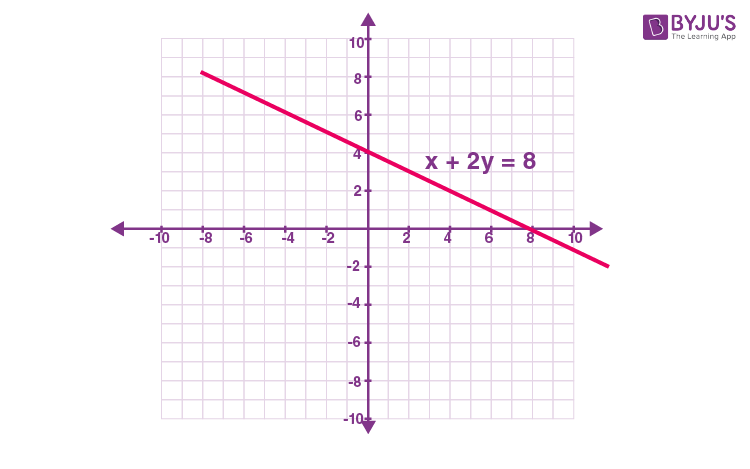Here, the graph of x + 2y = 8 is the line that is not passing through the point (-1, -2).

Hence, (-1, -2) is not a solution to this equation.

Question 14- Diagonals AC and BD of a quadrilateral ABCD intersect at O in such a way that ar(AOD) = ar(BOC). Prove that ABCD is a trapezium.

Solution:

Given,

ABCD is a quadrilateral in which AC and BD are diagonals which intersect at O.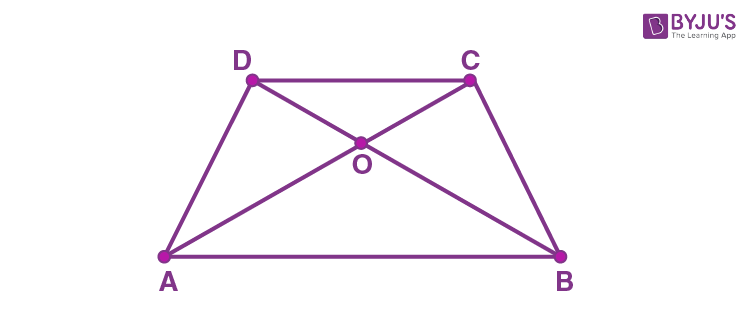Also, ar(∆AOD) = ar(∆BOC)

ar(∆AOD) + ar(∆AOB) = ar(∆BOC)+ ar(∆AOB)

⇒ ar(∆ABD) = ar(∆ABC)

Here, the triangles ABD and ABC are on the same base AB and have the same areas.

Therefore, they must lie in between the same parallels, i.e. AB and CD.

i.e. AB || DC

Hence, ABCD is a trapezium.

Question 15- If a+b+c = 0, then show that

$$\begin{array}{l}a^{4}+b^{4} + c^{4} = 2(b^{2}c^{2} + c^{2}a^{2}+ a^{2}b^{2})\end{array}$$

Solution:

Given,

a + b + c = 0

a + b = -c

Squaring on both sides,

(a + b)² = (-c)²

a²+ b² + 2ab = c²

a²+ b²  – c² = -2ab

Again squaring on both sides,

(a² + b² – c²)² = (-2ab)²

a⁴ + b⁴ + c⁴ + 2a²b² – 2b²c² – 2c²a² = 4a²b²

a⁴ + b⁴ + c⁴ + 2a²b² – 4a²b² – 2b²c² – 2c²a² = 0

a⁴ + b⁴ + c⁴ – 2a²b² – 2b²c² – 2c²a² = 0

a⁴ + b⁴ + c⁴ = 2a²b² + 2b²c² + 2c²a²

a⁴ + b⁴ + c⁴ = 2(a²b² + b²c² + c²a²)

Question 16- A solid cube has been cut into two cuboids of equal volumes. Find the ratio of the total surface area of one of the cuboids to that of the given cube.

Solution:

Let a be the edge of a solid cube.

TSA of cube = 6a²

Given that the cube is cut into two cuboid with equal volume.

Thus, the cube will be cut into two equal halves along its side.

Now, the dimensions of cuboid are:

Length (l) = a

Height (h) = a/2

TSA of cuboid = 2(lb + bh + lh)

= 2[a² + (a²/2) + (a²/2)]

= 2 (2a²)

= 4a²

Required ratio = (TSA of cuboid) : (TSA of cube)

= 4a² : 6a²

= 2 : 3

Question 17- A storage tank is in the form of a cube. When it is full of water, the volume of the water is 15.625

$$\begin{array}{l}m^{3}\end{array}$$
. If the present depth of the water is 1.3 m, find the volume of water already used from the tank.

Solution:

Let a be the side of a cube.

Given,

Volume of cubical tank = 15.625 m3

⇒  a3 = 15.625 m3

⇒  a = 2.5 m

Present depth of water in cubical tank = 1.3 m (given)

∴ Height of water used = (2.5 – 1.3) m = 1.2 m

Volume of water used = (1.2) × (2.5) × (2.5) = 7.5 m3

= 7. 5 x 1000

= 7500 L (since 1 m3 = 1000 L)

Therefore, the volume of water already used from the tank = 7500 L

Question 18- A spherical cannonball, 28 cm in diameter is melted into a right circular conical mould, the base of which is 35 cm in diameter. Find the height of the cone, correct to one place of decimal.

Solution:

Given,

Radius of spherical cannonball = 14 cm

Volume of cannon ball = 4/3 πr3

= (4/3) π(14)3

Diameter of base of a conical mould = 35 cm (given)

Radius of cone = 35/2 cm

Let h be the height of the cone.

Volume of cone = 1/3 πr²

= (1/3) π(35/2)² h

From the given,

Volume of cannonball = Volume of cone

(4/3) π (14)3  = (1/3) π(35/2)² h

h = 35.84

Therefore, the height of the cone is 35.8 cm (one decimal point).

Question 19- In a

$$\begin{array}{l}\Delta ABC\end{array}$$
, median AD is produced to X such that AD = DX. Prove that ABXC is a parallelogram.

Solution:

Given,

In ΔABC, median AD is produced to X such that AD = DX.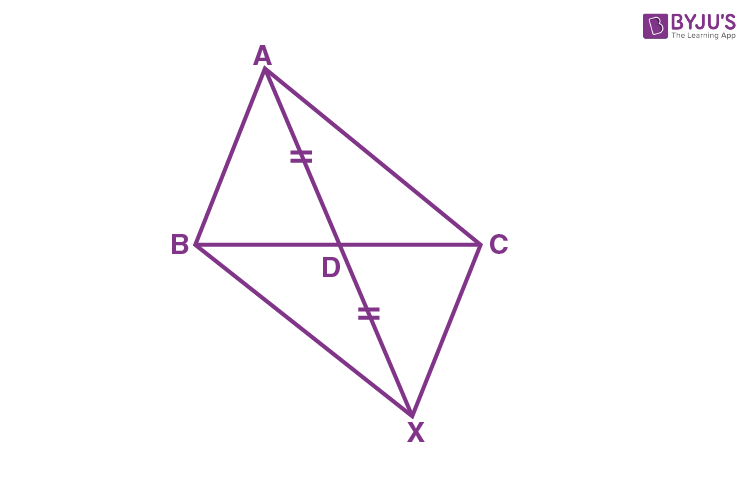BD = DC  (since AD is median)

That means, diagonals AX and BC bisect each other in quadrilateral ABXC.

Therefore, ABXC is a parallelogram.

Question 20- The force exerted to pull a cart is directly proportional to the acceleration produced in the body. Express the statement as a linear equation of two variables and draw the graph of the same by taking the constant mass equal to 6 kg. Also, find the force required when the acceleration produced is equal to

(i) 5

$$\begin{array}{l}m/sec^{2}\end{array}$$

(ii) 6

$$\begin{array}{l}m/sec^{2}\end{array}$$

(iii) 15

$$\begin{array}{l}m/sec^{2}\end{array}$$

Solution:

Given,

The force exerted to pull a cart is directly proportional to the acceleration produced in the body.

i.e. F = ma (here m is the proportionality constant and is also called mass of the body)

Constant mass (m) = 6 kg

That means, F =  6a

When a = 1, F = 6

When a = 2, F = 12

Let us draw the graph as shown below: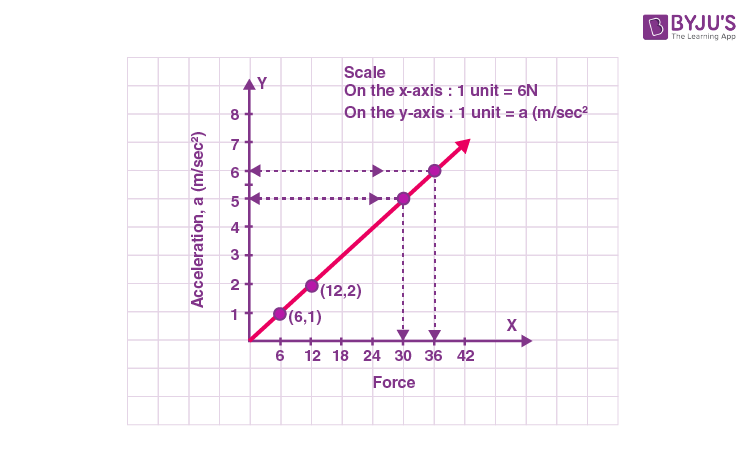(i) When the acceleration produced is equal to 5 m/sec², the force = 30 N

(ii) When the acceleration produced is equal to 6 m/sec², the force = 36 N

Similarly,

(iii) When the acceleration produced is equal to 15 m/sec², the force = 90 N (since F = 6a)